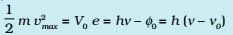The phenomena of interference, diffraction and polarization were explained in a natural and satisfactory way by the wave picture of light. According to this picture, light is an electromagnetic wave consisting of electric and magnetic fields with continuous distribution of energy over the region of space over which the wave is extended.

According to the wave picture of light, the free electrons at the surface of the metal (over which the beam of radiation falls) absorb the radiant energy continuously. The greater the intensity of radiation, the greater is the amplitude of electric and magnetic fields.

In this picture, the maximum kinetic energy of the photoelectrons on the surface is then expected to increase with increase in intensity.

### Einstein’s Photoelectric Equation: Energy Quantum of Radiation

Einstein’s photoelectric equation is in accordance with the energy conservation law as applied to the photon absorption by an electron in the metal. The maximum kinetic energy is equal to the photon energy (hν) minus the work function of the target metal:This photoelectric equation explains all the features of the photoelectric effect. Millikan’s first precise measurements confirmed the Einstein’s photoelectric equation and obtained an accurate value of Planck’s constant h. This value was close to the value of Planck’s constant (= 6.626 × 10-34J s). This led to the acceptance of particle or photon description (nature) of electromagnetic radiation, introduced by Einstein.